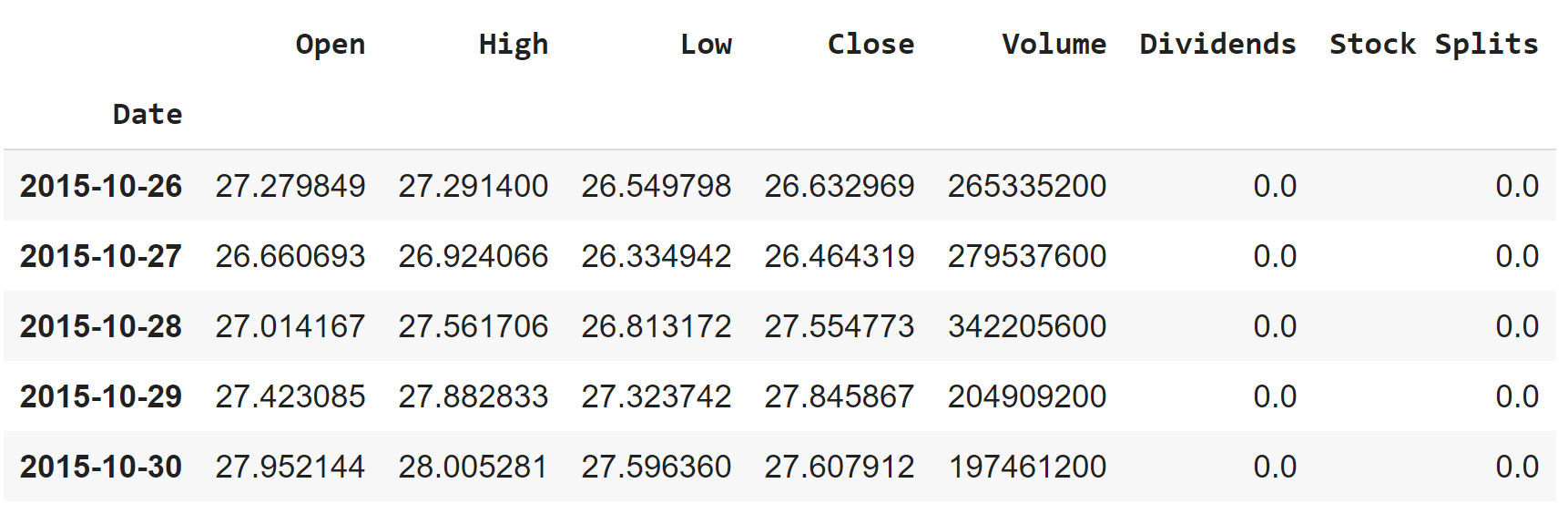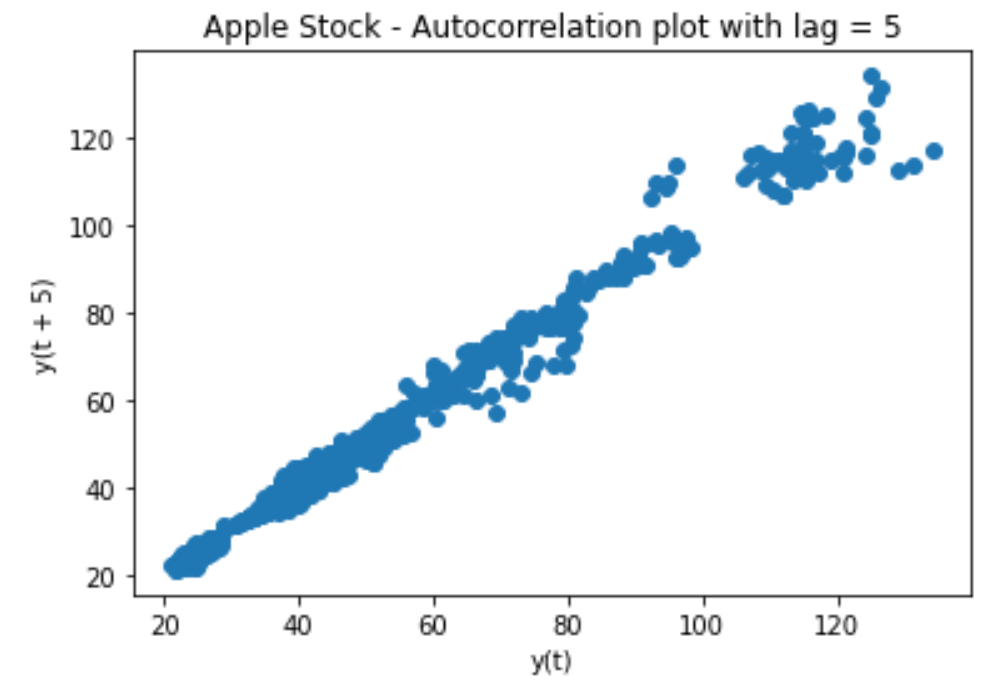### Timeseries Forcasting: Predicting Stocks using ARIMA model (Python)

Time Series Forecasting involves taking models fit on historical data and using them to predict future observations. Time series is used for non stationary data. Non Stationary data is the the data whose metrics like mean and standard deviation are not constant over time. These values keep on changing according to time. This non stationary data is refereed to as time series. Some examples of this is Stock prices over time, temperature over time and house prices overtime. In this post, we are going to do time series analysis using Apple stock and for this we are going to use ARIMA model.## What is ARIMA model?

ARIMA model or AutoRegressive Integrated Moving Average is one of the most famous and widely used forecasting method for time-series prediction. ARIMA models are capable of capturing a suite of different standard temporal structures in time-series data. ARIMA model explains a given time series based on its own past values, that is, its own lags and the lagged forecast errors, so that equation can be used to forecast future values. ARIMA model consist of three components which are:

• Auto Regressive (AR), which is the relationship between means that the model uses the dependent relationship between observation and some lagged observations. The parameter for this is p (number of lag observations).
• Integrated (I) which is the difference of raw observations, subtracting of the observation form a previous observation. This makes the time series more stationary. The parameter for this is d (degree of differencing).
• Moving Average (MA) which is the relationship between the residual error and the observations. The parameter for this is q (size of the moving average window).

## Analysis

We are using the Yahoo Finance API to get the data and we are going to predict the close values in this dataset. Lets start with a Python working example:

### Step 1: Load the libraries and data

import numpy as np
import yfinance as yf
import pandas as pd
import matplotlib.pyplot as plt
from pandas.plotting import lag_plot
from pandas import datetime
from statsmodels.tsa.arima_model import ARIMA
from sklearn.metrics import mean_squared_error
from statsmodels.tsa.seasonal import seasonal_decompose

aapl= yf.Ticker("aapl")
aapl_historical = aapl.history(period="5y", interval="1d")

This will get the data in aapl_historical variable for apple stock for 5 years at an interval of 1 day. It will look something like this.### Step 2: EDA

Now that we have loaded the libraries lets do some create some auto correlation plot to check if ARIMA can be performed on this data or not.

plt.figure()
lag_plot(aapl_historical['Close'], lag=5)
plt.title('Apple Stock - Autocorrelation plot with lag = 5')
plt.show()The auto correlation at a lag of 5 shows that these values are a related. Now lets check different components of this time series.

decomposition = seasonal_decompose(aapl_historical["Close"], freq=30)
trend = decomposition.trend
seasonal  = decomposition.seasonal
residual = decomposition.resid

plt.figure(figsize=(8,8))
plt.subplot(4,1,1)
plt.plot(aapl_historical["Close"], label = "Original")
plt.legend(loc = "best")
plt.subplot(4,1,2)
plt.plot(trend, label = "Trend")
plt.legend(loc = "best")
plt.subplot(4,1,3)
plt.plot(seasonal, label = "Seasonal")
plt.legend(loc = "best")
plt.subplot(4,1,4)
plt.plot(residual, label = "Residual")
plt.legend(loc = "best")
plt.tight_layout()
plt.show()